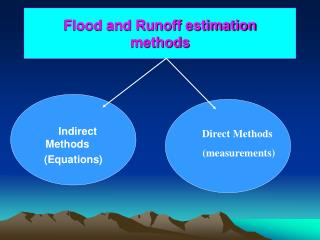DownloadDownload PresentationFlood and Runoff estimation methods

# Flood and Runoff estimation methods

Download Presentation## Flood and Runoff estimation methods

- - - - - - - - - - - - - - - - - - - - - - - - - - - E N D - - - - - - - - - - - - - - - - - - - - - - - - - - -
##### Presentation Transcript

1. Flood and Runoff estimationmethods Indirect Methods (Equations) Direct Methods (measurements)

2. Talbot’s CN (SCS) Rational Indirect Methods Unit Hydrograph Manning’s Formula EquationCreager’s

3. Direct Methods Current meter Staff gauge Crest stage gauge

4. SCS Method • SCS Method • In this method the runoff volume (Q) resulting from a given rainfall storm (P) is calculated using the following formula.

5. Talbot Method • Talbot Method • Design flood can be related to morphological properties of the catchment. Design flood discharge (Q), in m3 /sec, can be calculated using the following formula

6. Talbot Method • Talbot Method Runoff coefficients according to catchments characteristics

7. Estimation of Design Flood • Rational Method • The rational method uses existing rainfall data and land use in estimating peak runoff from small drainage areas that are less than 15 km2.

8. Estimation of Design Flood • Rational Method Runoff Coefficients for the Rational Method

9. Estimation of Design Flood • Rational Method • The rainfall intensity (i) in millimeters per hour for duration corresponding to the time of concentration for the catchment area and having a recurrence period appropriate to the site conditions, is read from IDF curves.

10. Estimation of Design Flood • Rational Method • Time of concentration is defined as the interval time in minutes from the beginning of rainfall to the time when water from the most remote position of the catchment reaches the outlet. • Kirkpich formula

11. Estimation of Design Flood • Rational Method

12. Estimation of Design Flood • SCS Method • In this method the runoff volume (Q) resulting from a given rainfall storm (P) is calculated using the following formula.

13. Estimation of Design Flood • Talbot Method • Design flood can be related to morphological properties of the catchment. Design flood discharge (Q), in m3 /sec, can be calculated using the following formula

14. Estimation of Design Flood • Talbot Method Runoff coefficients according to catchments characteristics

15. Estimation of Design Flood • Talbot Method Formulas according to catchment properties Q5 = 0.6 Q25; Q10 = 0.8Q25; Q50 = 1.2Q25; Q100 = 1.4Q25

16. CREAGER’S METHOD Where: Qm = Maximum or peak flow for a given return period. A = Catchment area (Sq. Km). C1 = Greagers number (max. 130).

17. Theoretical eq. for runoff est. for Yemen • المعادلات النظرية لاحتساب الجريان السطحي (لليمن): • Jac A.M. van der Gun and others 1995. R. O.= 0.055*P (mm). P= precipitation (mm) • Arnon 1972 R. O.= 0.6*P*S (mm). S=Slop • Runoff Coefficient Method V=103C.P.A (m3). C=Runoff Coefficient • Flood Estimation Q=C.I.A/3.6 (m3/s). I=Rainfall Intensity (mm/hr) • SCS Curve Number Method F/S = Q/Pe F=Actual water retention (Pe-Q) S=Potential Maximum Retention Q=Actual Runoff • Pe=Potential runoff

18. Flow measurements

19. Flow measurements

20. Flow measurementscurrent meters

21. Current meters